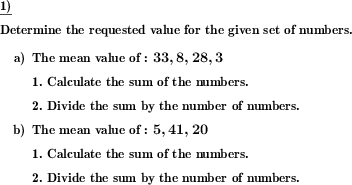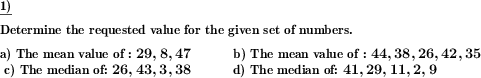Custom math worksheets at your fingertips# Details for problem "Mean and median to be determined"

Quickname: 3103

Elementary School, Primary School, Junior High School, Middle School, High School.

## Summary

For a series of numbers, the mean or median is to be found.

## Examples## Description

A series of whole numbers is presented. Depending on the task, the median or the mean value of the numbers is to be determined.

It can be set whether only the mean or only the median is asked for, or whether one of the two values is asked for in random alternation.

The number range from which the numbers are taken can be set. The count of the numbers in the numerical series can also be selected. If the median is asked for, it must be formed from the mean value of the two middle numbers if the number of numbers is even. To ensure that the median can always be read directly from the number series, it can be specified that the number of numbers is always odd when the median is asked for.

The number series is made so that the mean, like the numbers themselves, is always an integer.

If desired, the numbers can be presented sorted by value.

To simplify the task, the solution steps can be given in the task and/or in the solution.

These steps are provided as follows:

For the mean:

1. Calculate the sum of the numbers.

2. Divide the sum by the number of numbers.

For the median:

1. Order the numbers in order of their size.

2. For an even number of numbers: Calculate the median as the mean of the two middle numbers.

resp.

2. For an odd number of numbers: The number in the middle is the median.

The number of problems can be set.

Download free printable worksheets for this math problem here. The worksheet contains the problems only, the solution sheet includes the answers. Just click on the respective link.

•Worksheet 1Solution sheet with answers
•Worksheet 2Solution sheet with answers
•Worksheet 3Solution sheet with answers

If you can not see the solution sheets for download, they may be filtered out by an ad blocker that you may have installed. If this is the case, please allow ads for this page and reload the page. The solution sheets will then reappear.

• Do these sample worksheets do not really fit?
• Do you need more math worksheets, with a different level of difficulty?
• Would you like to combine different problems on a worksheet and adjust them to your needs?
• As a teacher, you can put together your own worksheets using the automatically generated math problems provided.
With a free initial credit, you can start creating your own math worksheets in a few minutes.

You can try it for free! Register here, to create custom worksheets now!

## Customization options for this problem

Parameter
Possible values
Number of problems
1, 2, 3, 4, 5, 6, 7, 8, 9, 10
Mixed, Median, Mean
Number of numbers
3-7, 7-9, 9-11
Even number of numbers allowed
Yes, No
Same number allowed multiple times
Yes, No
Sorted list of numbers given
Yes, No
Number range
1 - 50, 1 - 100, -20 - 20, -50 - 50, -100 - 100
Show solution steps
no, with solution, with problem&solution

## Similar problems

Remark
Description
Both median and mean are given as values, it has to be determined which value is what
For two given numbers for a number series, decide which is the median and which is the mean.
For a given value, decide whether it is the median or the mean value
Decide whether a number is the median or mean of a given series of whole numbers.Deutsche Version dieser Aufgabe
These informational pages with samples describe math problems that can be combined on custom math worksheets with solutions for home and K-12 school use.
Deutsche Seiten
×